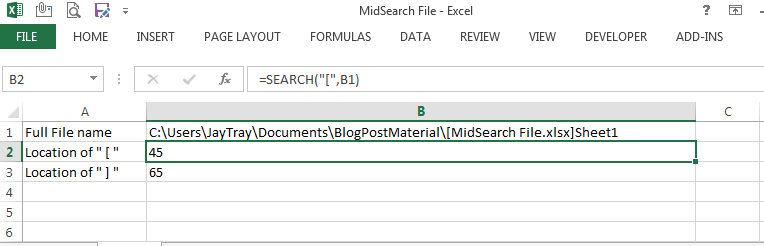# How to get filename in ExcelAs a follow on from the last post, I was asked how to get filename in Excel using similar formulas.

This can be done with the formula below.

=MID(CELL(“filename”),(SEARCH(“[“,CELL(“filename”))+1),(SEARCH(“]”,CELL(“filename”))-6-(SEARCH(“[“,CELL(“filename”)))))

Really simple isn’t it?  I bet you were wondering how you didn’t figure it out yourself!!

This combines 3 formulas, MID, CELL, and SEARCH.

I’ll go through each of the 3  formulas and how they are used together to get the result we want (the file name).

Before continuing though, I would advise you to read this post on brackets and commas if you haven’t already done so.  Understanding brackets and commas is very important when it comes to combining formulas like this, it makes them easier to read.

## Why do I want to know how to get filename in Excel?

You’re probably wondering why does a person want to know how to get filename in Excel?  The reason I use it, and I believe it is the same for the person that was asking me for this, is to use the filename as a variable when writing VBA macros that switch between several files.

If you’ve no interest in knowing how to get filename in Excel, or VBA macros, that doesn’t mean you may have no interest in the rest of this post.

It can be good practice for combining formulas together and for understanding long formulas which may look daunting and complicated.

## CELL

The CELL formula returns information about the cell the formula is in.  As you type the formula, after you open the brackets you may see a list of options you can select from.

In our case, we want to retrieve the filename.

Our formula is =CELL(“filename”) and this gives us the full file details of our cell including the directory/folder the file is saved in, the file name and the sheet name.From the very top of the screen, you can see the file name is “MidSearch File” and within the result of our formula you can see the filename is contained between two brackets [ and ].

We will look to pull the filename from this formula result.

## SEARCH

The SEARCH formula searches for a character and returns it’s character number or location within selected text.

We are going to use the SEARCH formula to find the location of the [ and ] brackets which surround our filename within our result from the CELL formula.

The CELL formula is in cell B1, this is the text that we will be querying.

The syntax of the SEARCH formula is =SEARCH(find_text, within_text, [start_num]).  We will only be using the first two sections, which are:

• find_text : we will be looking for “[“
• within_text : we are going to search within the text in cell B1, which is the result of our CELL formula.

Our formula is =SEARCH(“[“,B1).This tells us that in our full filename, the “[” is the 45th character.  So, our filename begins from the 46th character.

If we use the same formula searching for “]” our formula is =SEARCH(“]”,B1) and the result tells us that this is the 65th character of our CELL result.

So, our formula ends with the 59th character, which is the 65th character minus 6.  The 6 that we want to ignore end up being “.xlsx]”.

## MID

The last post covered the MID formula.

The syntax is =MID(text, start_num, num_chars) and our formula is going to be made up of

• text : B1 – the result of our CELL formula in cell B1
• start_num : 46 – we have already established that the character number of “[” is 45, so we are going to start from the next character which is 46.
• num_chars : 14 – there are 14 characters in our filename.  I have counted these, but in the full formula we will use formulas to work this out.

The formula is =MID(B1, 46, 14).

…and hey presto, we have our filename without the sheetname and folder directory of the file.

So how do we put this all together?

## Combining Formulas

=MID(CELL(“filename”),(SEARCH(“[“,CELL(“filename”))+1),(SEARCH(“]”,CELL(“filename”))-6-(SEARCH(“[“,CELL(“filename”)))))

This long-winded formula is really just a MID formula that contains SEARCH and CELL formulas.

To refresh, the MID syntax is =MID(text, start_num, num_chars) and our formula broken down into its 3 parts is:

### TEXT

The text we are going to query is the full filename.  This is CELL(“filename”).

### START_NUM

Our starting point is going to be the first character after the “[“.  We use the SEARCH formula to search for [ in our filename and then go to the next location – basically the search result plus 1.  This is (SEARCH(“[“,CELL(“filename”))+1).

### NUM_CHARS

We need to work out how many characters are in our filename.

Two search formulas will be used, we get the position of “]” which is 65 and deduct the position of “‘[” which is 45.

This gives us 20 characters so far, but we also want to exclude “.xlsx]” so we deduct a further 6.

In english this is the character number of “[“, minus 6, minus the character number of  “]”.

The formula is (SEARCH(“]”,CELL(“filename”))-6-(SEARCH(“[“,CELL(“filename”)))).

### FULL FORMULA

The full formula again is  =MID(CELL(“filename”),(SEARCH(“[“,CELL(“filename”))+1),(SEARCH(“]”,CELL(“filename”))-6-(SEARCH(“[“,CELL(“filename”))))).Have you managed to breakdown the formula yourself into its individual formulas?

Are you able to replicate the formula yourself?

If you are ever unsure, or not confident you can type different formulas into different cells and then use those results to get the filename, almost like I have done in rows 1 to 4 above.

## Is there another way?

The short answer is…… probably.

This way works, it may look complicated but is really just a few formulas together.  Build up the full formula using the individual formulas and this can be put together surprisingly quickly.